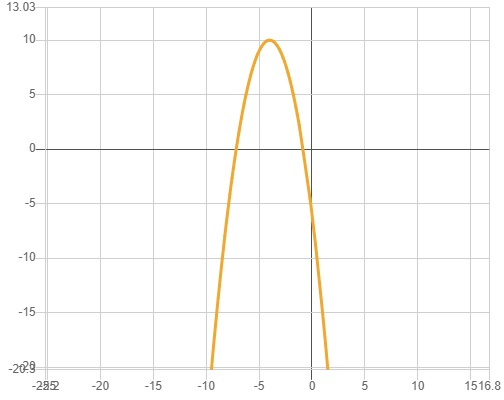elvishwitchxyp

2021-12-31

Find the following to accurately graph the quadratics below:
a) Table of 5 values (leave any non-whole numbers as fractions if not a terminating decimal)
b) The vertex (tell whether max/min.)
c) The y- intercept
$y=-{x}^{2}-8x-6$Virginia Palmer

Step 1
Since the given $y=-{x}^{2}-8x-6$
for x-intercepts, $y=6$
$-{x}^{2}-8x-6=0=1{x}^{2}+8x+6=0$
$\left(a{x}^{2}+bx+c=0\right)$
$\left(x=\frac{-b±\sqrt{{b}^{2}-4ac}}{2a}\right)$
$x=\frac{-8±\sqrt{64-24}}{2}$
$x=\frac{-8±\sqrt{40}}{2}$
$x=\frac{-8+\sqrt{40}}{2}$
$\frac{-8-\sqrt{40}}{2}=1x=\frac{-8+6.324}{2}$
$\frac{-8-6.324}{2}$
$x=-0.838,\phantom{\rule{1em}{0ex}}-7.162$
So, curse cuts x-axis at at
for y-intercepts $x=0$
$y=-6$
So, curse cuts y-axis at
$y=-\left({x}^{2}+8x+6\right)=-\left({x}^{2}+2×x×4+{\left(4\right)}^{2}-{\left(4\right)}^{2}+6\right)$
$y=-\left({\left(x+4\right)}^{2}-16+6\right)=-\left({\left(x+4\right)}^{2}-10\right)×-{\left(x+4\right)}^{2}+10$
$y=a{\left(h-h\right)}^{2}k$
So,
So vertex is
Now, make table
$\begin{array}{|ccccccccccc|}\hline x& 2& 4& 6& 8& 10& -2& -4& -6& -8& -10\\ y& -26& -54& -90& -184& 6& 10& 6& 6& -26\\ \hline\end{array}$Ronnie Schechter

Step 1
Given $y=-{x}^{2}-8x-6$
Swap sides so that all variable terms are on the left hand side.
$-{x}^{2}-8x-6=y$
Subtract y from both sides.
$-{x}^{2}-8x-6-y=0$
All equations of the form $a{x}^{2}+bx+c=0$ can be solved using the quadratic formula: $\frac{-b±\sqrt{{b}^{2}-4ac}}{2a}$. The quadratic formula gives two solutions, one when $±$ is addition and one when it is subtraction.
$-{x}^{2}-8x-y-6=0$
This equation is in standard form: $a{x}^{2}+bx+c=0$. Substitute -1 for a, -8 for b, and $-6-y$ for c in the quadratic formula, $\frac{-b±\sqrt{{b}^{2}-4ac}}{2a}$
$x=\frac{-\left(8\right)±\sqrt{{\left(-8\right)}^{2}-4\left(-1\right)\left(-y-6\right)}}{2\left(-1\right)}$
Square -8.
$x=\frac{-\left(-8\right)±\sqrt{64-4\left(-1\right)\left(-y-6\right)}}{2\left(-1\right)}$
Multiply -4 times -1
$x=\frac{-\left(-8\right)±\sqrt{64+4\left(-y-6\right)}}{2\left(-1\right)}$
Multiply 4 times $-6-y$
$x=\frac{-\left(8\right)±\sqrt{64-4y-24}}{2\left(-1\right)}$
Add 64 to $-24-4y$
$x=\frac{-\left(-8\right)±\sqrt{40-4y}}{2\left(-1\right)}$
Take the square of $40-4y$
$x=\frac{-\left(-8\right)±2\sqrt{10-y}}{2\left(-1\right)}$
The opposite of -8 is 8
$x=\frac{8±2\sqrt{10-y}}{2\left(-1\right)}$
Multiply 2 times -1
$x=\frac{8±2\sqrt{10-y}}{-2}$
Now solve the equation $x=\frac{8±2\sqrt{10-y}}{-2}$ when $±$ is plus. Add 8 to $2\sqrt{10-y}$
$x=\frac{2\sqrt{10-y}+8}{-2}$
Divide $8+2\sqrt{10-y}$ by -2
$x=-\left(\sqrt{10-y}+4\right)$
When $±$ is minus. Subtract